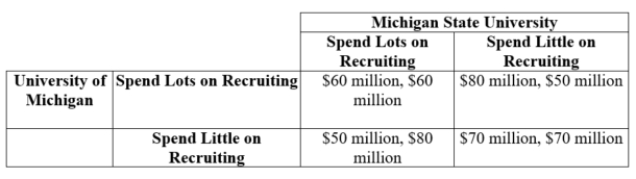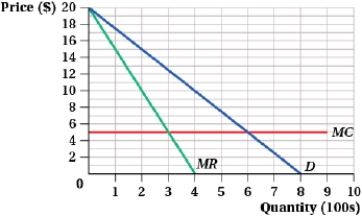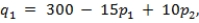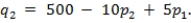# Microeconomics Study Set 46

## Quiz 11 : Imperfect CompetitionStudy FlashcardsLooking for Economics Homework Help?

## Quiz 11 :Imperfect Competition

Question Type(Table: College Football Recruitment) The table shows the payoffs associated with two levels of spending for recruitment of star football players. Payoffs: University of Michigan's Football Revenue, Michigan State's Football RevenueWhat is the Nash equilibrium?
Free
Multiple Choice

B

Tags
Choose question tagIn a Bertrand competition, with differentiated goods in a market structure of two firms, firm A's first-order condition can be expressed as:
Free
Multiple Choice

A

Tags
Choose question tagThe inverse demand for shampoo is given by P = 30 - 0.03Q, where P is the price per bottle in dollars and Q is bottles brought to market in hundreds. There are two manufacturers in the local market. Firm 1's cost function is given by C1 = 0.05q12, where q1 is the number of bottles it brings to market. Firm 2's cost function is given by C2 = 0.03q22, where q2 is the number of bottles it brings to market. The two firms are Cournot competitors who set output so that Q = q1 + q2. In equilibrium, the market quantity is ____.
Free
Multiple Choice

D

Tags
Choose question tagTwo firms are producing identical goods in a market characterized by the inverse demand curve P = 60 - 2Q, where Q is the sum of Firm 1 and Firm 2's output, q1 + q2. Each firm's marginal cost is constant at $12, and fixed costs are zero. Answer the following questions, assuming that the firms are Cournot competitors. In this case, Firm 2 earns$____ in profit.
Multiple Choice
Tags
Choose question tagThe market inverse demand curve for thrust bearings is P = 15 - 1.5Q, where Q is measured in hundreds of bearings per day and P is the price per bearing. The marginal cost is $3. Suppose two firms, which are Bertrand competitors, produce identical thrust bearings for this market. If this market were monopolized, the market price would be$____.
Multiple Choice
Tags
Choose question tagTwo companies are the only snowplow merchants in a small town. Inverse market demand curve is P = 100 - 10Q, where Q = q1 + q2 (Firm 1's output = q1; Firm 2's output = q2). Each firm has marginal costs of $25. In the Nash equilibrium in this market, Firm 2 produces ____. Multiple Choice Answer: Tags Choose question tagIn a Cournot market structure with two firms, firm A's reaction function gives: Multiple Choice Answer: Tags Choose question tagThe inverse demand for tacos is given by P = 10 - 0.02Q, where P is the price per taco and Q is the total number of tacos brought to market. There are two taco shops in the local market. Shop 1's cost function is given by C1 = 0.01q12, where q1 is the number of tacos it brings to market. Shop 2's cost function is given by C2 = 0.01q22, where q2 is the number of tacos it brings to market. Assume the two shops compete by setting output (Cournot). Let Q = q1 + q2. In equilibrium, the market price is$____.
Multiple Choice
Tags
Choose question tag(Figure: Market for Two-Firm Industry I) The graph depicts the market demand curve for a two-firm industry.If the two firms collude and evenly split the market output, how much output will each firm produce?
Multiple Choice
Tags
Choose question tagTwo firms that are engaged in Stackelberg competition face the market inverse demand curve P = 100 - 2Q, where Q is the total market output comprising Firm 1's output, q1, and Firm 2's output, q2. Each firm produces the product at a constant marginal cost of $22. If Firm 2's reaction function is q2 = 22 - 0.5q1, what is Firm 1's (the first-mover's) inverse demand curve? Multiple Choice Answer: Tags Choose question tagIn monopolistic competition, the long-run equilibrium price _____ marginal cost because _____. Multiple Choice Answer: Tags Choose question tagGotcha, the only seller of stun guns, faces the inverse market demand curve P = 400 - 12Q, where Q measures the number of stun guns per day and P is the price per stun gun. The marginal cost is constant at$64. Gotcha's profit-maximizing quantity is ____.
Multiple Choice
Tags
Choose question tagThe inverse demand for designer blankets is given by P = 40 - 0.01Q, where P is the price per blanket and Q is the total number of blankets brought to market. Two shops in the market supply specialty blankets. Shop 1's cost function is given by C1 = 0.02q12, where q1 is the number it brings to market. Shop 2's cost function is given by C2 = 0.02q22, where q2 is the number it brings to market. Given that the two shops compete by setting output (Cournot), the profit maximizing level of output for Shop 2 is ____.
Multiple Choice
Tags
Choose question tagTwo firms are producing identical goods in a market characterized by the inverse demand curve P = 60 - 2Q, where Q is the sum of Firm 1 and Firm 2's output, q1 + q2. Each firm's marginal cost is constant at $12, and fixed costs are zero. Answer the following questions, assuming that the firms are Cournot competitors. In this case, the market price is$____.
Multiple Choice
Tags
Choose question tagSuppose that Etsy (an e-commerce site focused on handmade or vintage items) necklace vendors compete in a Bertrand market structure with differentiated products. Demand for style 1, produced by vendor 1, is given bywhere p1 is price of style 1 and p2 is the price of style 2, produced by vendor 2. Demand for style 2 isThe costs of providing these necklaces are C1 = q1 and C2 = 0.75q2 respectively. The equilibrium price for style 2 is $____. Multiple Choice Answer: Tags Choose question tagThe inverse demand for shampoo is given by P = 30 - 0.03Q, where P is the price per bottle in dollars and Q is bottles brought to market in hundreds. There are two manufacturers in the local market. Firm 1's cost function is given by C1 = 0.05q12, where q1 is the number of bottles it brings to market. Firm 2's cost function is given by C2 = 0.03q22, where q2 is the number of bottles it brings to market. The two firms are Cournot competitors who set output so that Q = q1 + q2. In equilibrium, the market price is$____.
Multiple Choice
Tags
Choose question tagSuppose that Mystic Energy and E-Storm are the only two producers of hydrogen fuel cells. The market inverse demand curve for hydrogen fuel cells is P = 1,300 - 0.08Q, where Q is the number of fuels cells per month and P is the price per fuel cell. The marginal cost is constant at $500. Acting as a cartel, the owners of Mystic Energy and E-Storm agree to evenly split the market output. In this case, E-Storm earns a profit of$ ____.
Multiple Choice
Tags
Choose question tagNey Inc. and ARN Parts are the only two producers of bulldozer bucket teeth. The owners of the two firms conspire to charge a monopoly price, with each firm serving half the market. The market inverse demand curve is P = 1,000 - 10Q, where Q measures the daily number of sets of bulldozer bucket teeth and P is the price per set. The marginal cost of production for either firm is constant at $200, and fixed costs are zero. The profit for Ney Inc. is$_____.
Multiple ChoiceThe market inverse demand curve for thrust bearings is P = 15 - 1.5Q, where Q is measured in hundreds of bearings per day and P is the price per bearing. The marginal cost is $3. Suppose two firms, which are Bertrand competitors, produce identical thrust bearings for this market. If this market were monopolized, the market quantity would be ____. Multiple Choice Answer: Tags Choose question tagThe market inverse demand curve for thrust bearings is P = 15 - 1.5Q, where Q is measured in hundreds of bearings per day and P is the price per bearing. The marginal cost is$3. Suppose two firms, which are Bertrand competitors, produce identical thrust bearings for this market. In this case, the market price is \$____.